# Mecon (truncated octahedron) Tiles

Written by Paul Bourke
Inspired by Nascif Abousalh-Neto
April 2020

ps: The name "mecon" was given to the truncated octahedron by Buckminster Fuller.

In the following, 2D tiling patterns will be generated by slicing a space-filling tiling in 3D space constructed from truncated octahedrons. The truncated octahedron being one of only five space-filling convex polyhedra with regular faces, the others are the triangular prism, hexagonal prism and of course the cube.

The images, geometry, renderings here were created with PovRay. But independent of which software is used, CSG (constructive solid geometry) models of the truncated octahedron are required, otherwise slicing polygonal models will not result in solid interiors. One way to create a truncated octahedron starting from a solid cube might be to first shave off the cube to form an octahedron (8 cuts required) and then shave off the octahedron to form the truncated octahedron (6 further cuts required). So, a total of 14 cuts, each one corresponding to a face of the final truncated octahedron. The PovRay formulation is given below but the details are also relevant for construction in other CSG software.

```// Create octahedron from a cube
// This is 2 units high (1 unit from origin to peak)
// and sqrt(2)/2 units wide, to center of edge on x-y plane
// and distance to any vertex is 1
#declare OTHETA = degrees(atan(sqrt(2))); // 54.7356103157 degrees
#declare OWIDTH = sqrt(2)/2;
#declare octahedron = difference {
box { <-1,-1,-1>,<1,1,1> }
plane { <0,0,-1>, 0 rotate <0,OTHETA,0> translate <0,0,1> }
plane { <0,0,-1>, 0 rotate <0,OTHETA,0> rotate <0,0,90> translate <0,0,1> }
plane { <0,0,-1>, 0 rotate <0,OTHETA,0> rotate <0,0,180> translate <0,0,1> }
plane { <0,0,-1>, 0 rotate <0,OTHETA,0> rotate <0,0,-90> translate <0,0,1> }
plane { <0,0,1>, 0 rotate <0,-OTHETA,0> translate <0,0,-1> }
plane { <0,0,1>, 0 rotate <0,-OTHETA,0> rotate <0,0,90> translate <0,0,-1> }
plane { <0,0,1>, 0 rotate <0,-OTHETA,0> rotate <0,0,180> translate <0,0,-1> }
plane { <0,0,1>, 0 rotate <0,-OTHETA,0> rotate <0,0,-90> translate <0,0,-1> }
}

// Create truncated octahedron from the octahedron
#declare truncoctahedron = difference {
object { octahedron }
plane { <0,0,-1>, 0 translate <0,0,2/3> } // top
plane { <0,0,1>, 0 rotate <0,90,0> rotate <0,0,45> translate <-2*OWIDTH/3,-2*OWIDTH/3,0> }
plane { <0,0,1>, 0 rotate <0,90,0> rotate <0,0,-45> translate <-2*OWIDTH/3,2*OWIDTH/3,0> }
plane { <0,0,-1>, 0 rotate <0,90,0> rotate <0,0,45> translate <2*OWIDTH/3,2*OWIDTH/3,0> }
plane { <0,0,-1>, 0 rotate <0,90,0> rotate <0,0,-45> translate <2*OWIDTH/3,-2*OWIDTH/3,0> }
plane { <0,0,1>, 0 translate <0,0,-2/3> } // bottom
}
```Grey shows the octahedron before slicing off each "corner vertex".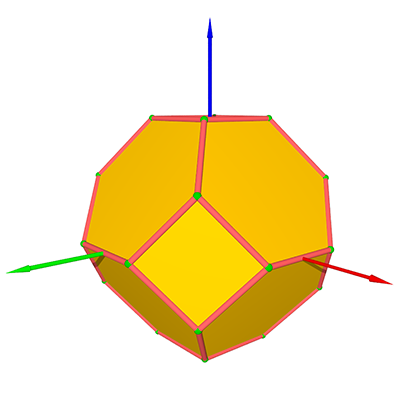Truncated octahedron in gold

This can be simplified by noticing that the square faces of the truncated octahedron are in a parallel plane to the faces of the cube, if it is rotated by 45 degrees from the axis aligned cube used above. So one can start with a smaller cube and create the truncated octahedron directly (total of 8 cuts required).

```// Create truncated octahedron directly from a cube
// This is 2 units high (1 unit from origin to peak)
// Square faces along coordinate system planes
#declare OTHETA = degrees(atan(sqrt(2))); // 54.7356103157 degrees
#declare truncoctahedron = difference {
box { <-1,-1,-1>,<1,1,1> scale 2/3 rotate <0,0,45> }
plane { <0,0,-1>, 0 rotate <0,OTHETA,0> translate <0,0,1> }
plane { <0,0,-1>, 0 rotate <0,OTHETA,0> rotate <0,0,90> translate <0,0,1> }
plane { <0,0,-1>, 0 rotate <0,OTHETA,0> rotate <0,0,180> translate <0,0,1> }
plane { <0,0,-1>, 0 rotate <0,OTHETA,0> rotate <0,0,-90> translate <0,0,1> }
plane { <0,0,1>, 0 rotate <0,-OTHETA,0> translate <0,0,-1> }
plane { <0,0,1>, 0 rotate <0,-OTHETA,0> rotate <0,0,90> translate <0,0,-1> }
plane { <0,0,1>, 0 rotate <0,-OTHETA,0> rotate <0,0,180> translate <0,0,-1> }
plane { <0,0,1>, 0 rotate <0,-OTHETA,0> rotate <0,0,-90> translate <0,0,-1> }
scale 3/2
rotate <0,0,-45>
}
```

The 3 orthogonal axis aligned views are illustrated below: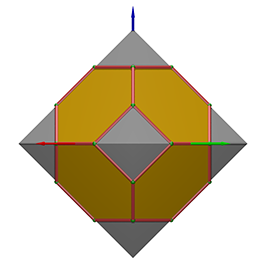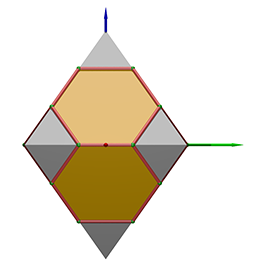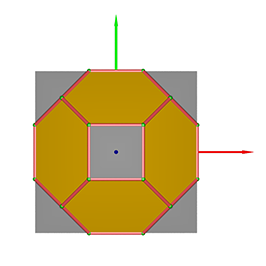The tiling of 3D space consists of two intertwined lattices as shown below. One lattice consists of the original truncated octahedral cell above displaced by integer multiples of (2,0,0), (0,2,0) and (0,0,2). The second lattice uses the same displacements but for the original truncated octahedral cell displaced by (1,1,1).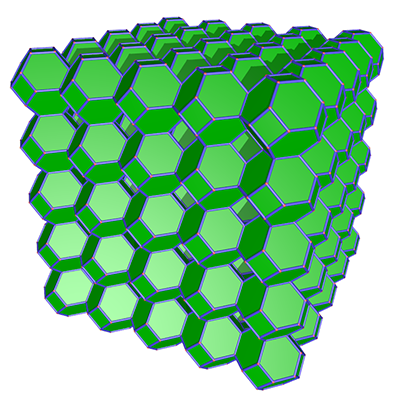Combined.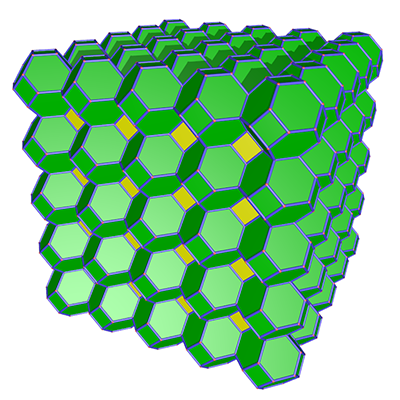A frame around the truncated octahedral cell will make the tiling boundaries clearer. This is achieved in CSG by "chocolate coating" the truncated octahedron cell. Specifically, take the mecon CGS cell and give it a black texture, subtract a slightly smaller version of the cell, and then place the same smaller cell, with colour of choice, in the interior. In CSG terms this might be described as follows.

```#declare chocolatecoated = union {
object {
truncoctahedron scale 0.98
texture { texture1 }
}
difference {
object {
truncoctahedron
texture { theblack }
}
object {
truncoctahedron scale 0.98
texture { texture1 }
}
}
}
```

Various cutting planes are presented below.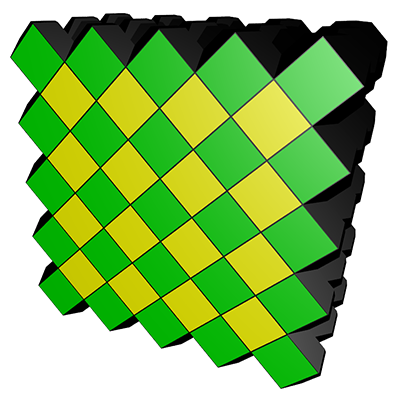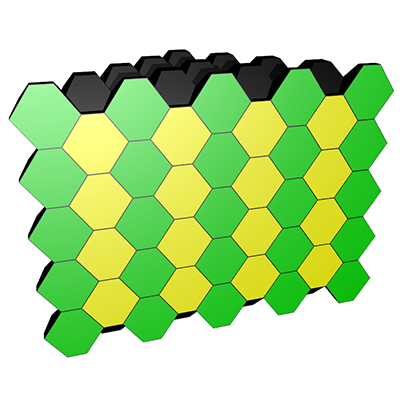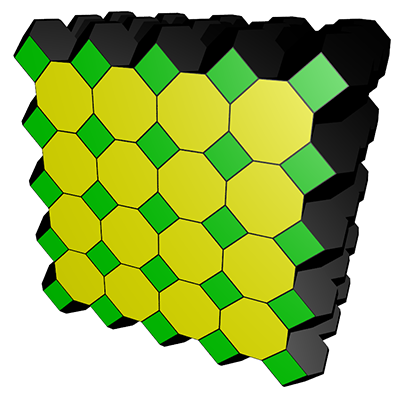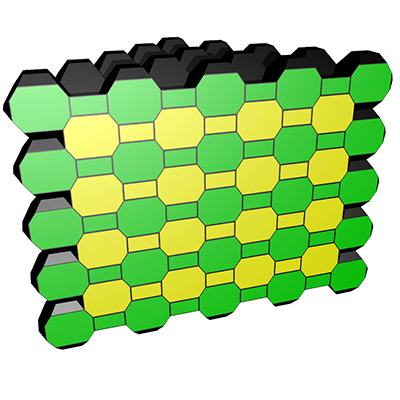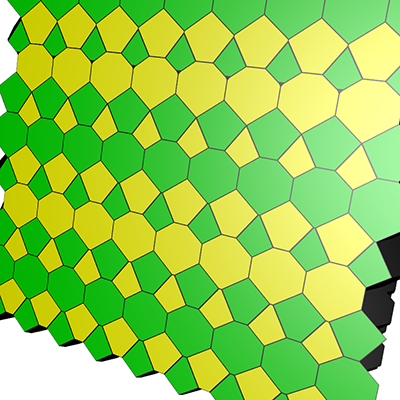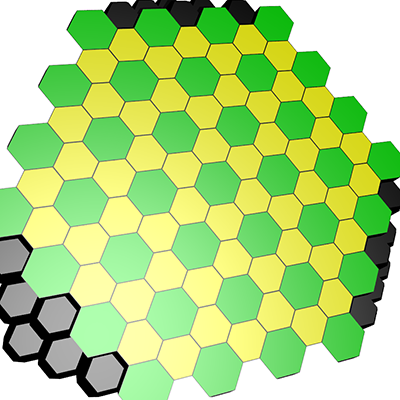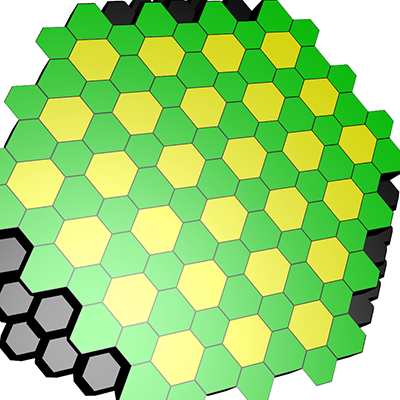One can of course create "new" 3D tilings by extracting cubic regions from the truncated octahedral tiling.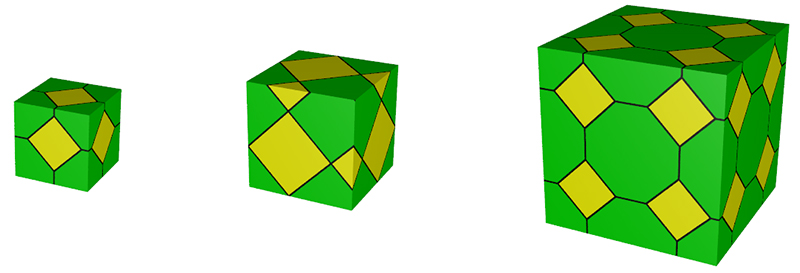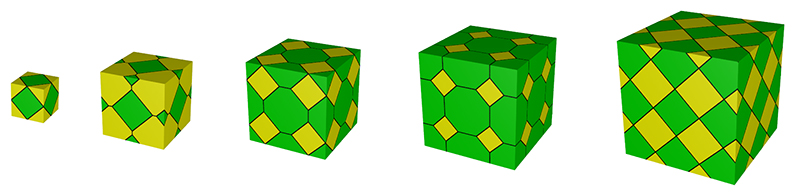... or discover spherical tesselations.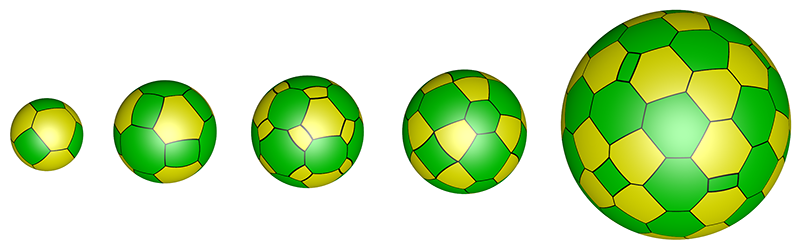View from the interior as an equirectangular projection.Navigable versionNavigable version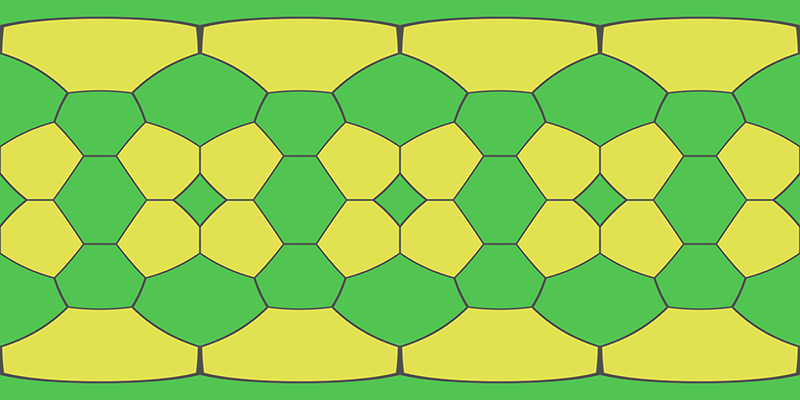Navigable version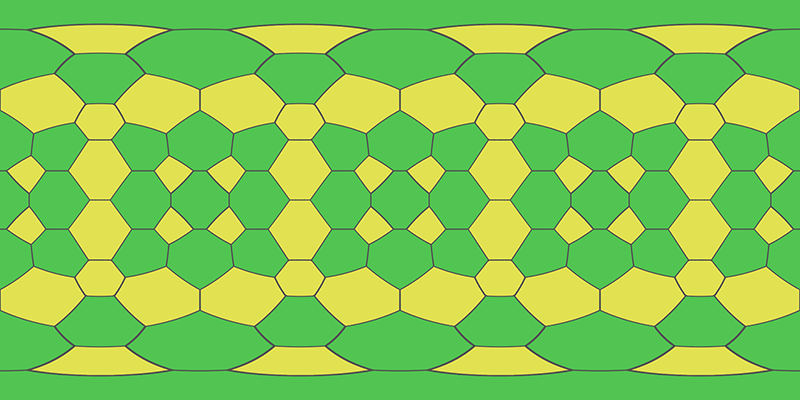Navigable version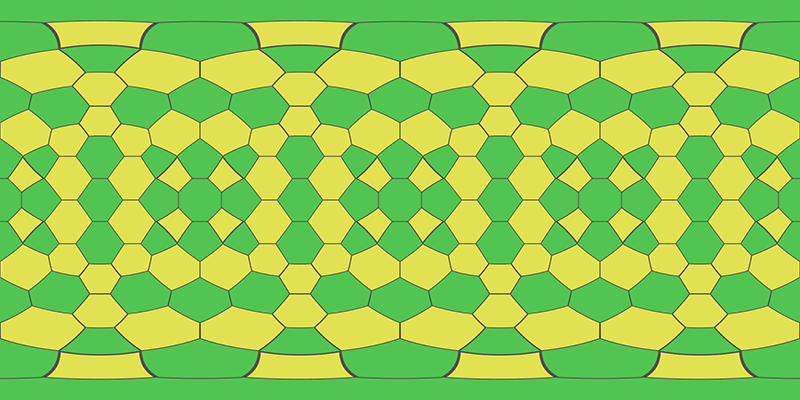Navigable version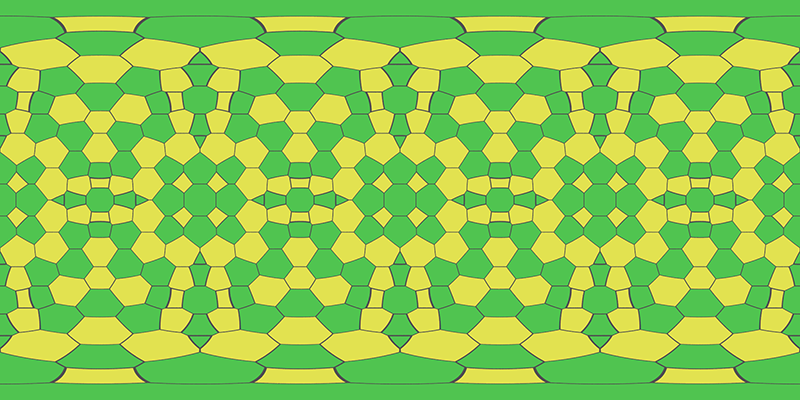Navigable version

For those who may wish to explore vertex/edge molels, here are the vertices and edges for the truncated octahedron. We define the following.

 A1 = (cos( 45)/3,sin( 45)/3,2/3) A2 = (cos(135)/3,sin(135)/3,2/3) A3 = (cos(225)/3,sin(225)/3,2/3) A4 = (cos(315)/3,sin(315)/3,2/3) AM1 = (cos( 45)/3,sin( 45)/3,-2/3) AM2 = (cos(135)/3,sin(135)/3,-2/3) AM3 = (cos(225)/3,sin(225)/3,-2/3) AM4 = (cos(315)/3,sin(315)/3,-2/3) C1 = (cos( 45),sin( 45),0) C2 = (cos(135),sin(135),0) C3 = (cos(225),sin(225),0) C4 = (cos(315),sin(315),0)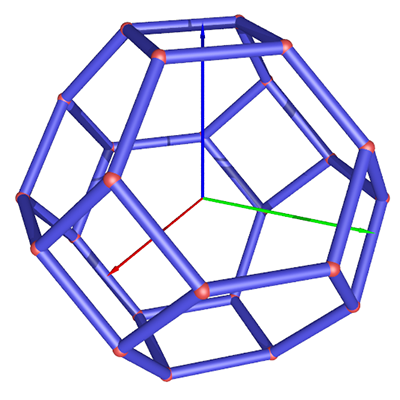The list of 24 vertices are:

A1, A2, A3, A4, AM1, AM2, AM3, AM4,
C1+(C2-C1)/3, C1+2*(C2-C1)/3, C2+(C3-C2)/3, C2+2*(C3-C2)/3,
C3+(C4-C3)/3, C3+2*(C4-C3)/3, C4+(C1-C4)/3, C4+2*(C1-C4)/3,
(A1 + C1)/2, (A2 + C2)/2, (A3 + C3)/2, (A4 + C4)/2,
(AM1 + C1)/2, (AM2 + C2)/2, (AM3 + C3)/2, (AM4 + C4)/2

The 36 edges are

A1 - A2
A2 - A3
A3 - A4
A4 - A1
AM1 - AM2
AM2 - AM3
AM3 - AM4
AM4 - AM1
A1 - (A1 + C1)/2
A2 - (A2 + C2)/2
A3 - (A3 + C3)/2
A4 - (A4 + C4)/2
AM1 - (AM1 + C1)/2
AM2 - (AM2 + C2)/2
AM3 - (AM3 + C3)/2
AM4 - (AM4 + C4)/2
(A1 + C1)/2 - C1+(C2-C1)/3
(A1 + C1)/2 - C4+2*(C1-C4)/3
(AM1 + C1)/2 - C1+(C2-C1)/3
(AM1 + C1)/2 - C4+2*(C1-C4)/3
(A2 + C2)/2 - C1+2*(C2-C1)/3
(A2 + C2)/2 - C2+(C3-C2)/3
(AM2 + C2)/2 - C1+2*(C2-C1)/3
(AM2 + C2)/2 - C2+(C3-C2)/3
(A3 + C3)/2 - C2+2*(C3-C2)/3
(A3 + C3)/2 - C3+(C4-C3)/3
(AM3 + C3)/2 - C2+2*(C3-C2)/3
(AM3 + C3)/2 - C3+(C4-C3)/3
(A4 + C4)/2 - C3+2*(C4-C3)/3
(A4 + C4)/2 - C4+(C1-C4)/3
(AM4 + C4)/2 - C3+2*(C4-C3)/3
(AM4 + C4)/2 - C4+(C1-C4)/3
C1+(C2-C1)/3 - C1+2*(C2-C1)/3
C2+(C3-C2)/3 - C2+2*(C3-C2)/3
C3+(C4-C3)/3 - C3+2*(C4-C3)/3
C4+(C1-C4)/3 - C4+2*(C1-C4)/3

The 12 faces (6 squares, 8 hexagons) are

A1 - A2 - A3 - A4
AM1 - AM2 - AM3 - AM4
(A2+C2)/2 - C2+(C3-C2)/3 - (AM2+C2)/2 - C1+2*(C2-C1)/3
(A3+C3)/2 - C3+(C4-C3)/3 - (AM3+C3)/2 - C2+2*(C3-C2)/3
(A4+C4)/2 - C4+(C1-C4)/3 - (AM4+C4)/2 - C3+2*(C4-C3)/3
(A1+C1)/2 - C4+2*(C1-C4)/3 - (AM1 + C1)/2 - C1+(C2-C1)/3
A1 - (A1+C1)/2 - C1+(C2-C1)/3 - C1+2*(C2-C1)/3 - (A2+C2)/2 - A2
A3 - A2 - (A2+C2)/2 - C2+(C3-C2)/3 - C2+2*(C3-C2)/3 - (A3+C3)/2
A4 - A3 - (A3+C3)/2 - C3+(C4-C3)/3 - C3+2*(C4-C3)/3 - (A4+C4)/2
A1 - A4 - (A4+C4)/2 - C4+(C1-C4)/3 - C4+2*(C1-C4)/3 - (A1+C1)/2
AM1 - AM2 - (AM2+C2)/2 - C1+2*(C2-C1)/3 - C1+(C2-C1)/3 - (AM1+C1)/2
AM2 - AM3 - (AM3+C3)/2 - C2+2*(C3-C2)/3 - C2+(C3-C2)/3 - (AM2+C2)/2
AM4 - AM3 - (AM3+C3)/2 - C3+(C4-C3)/3 - C3+2*(C4-C3)/3 - (AM4+C4)/2
AM1 - AM4 - (AM4+C4)/2 - C4+(C1-C4)/3 - C4+2*(C1-C4)/3 - (AM1+C1)/2